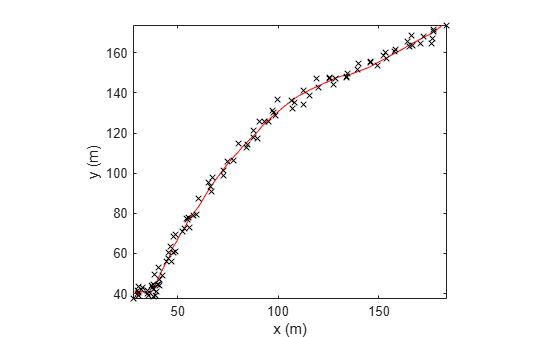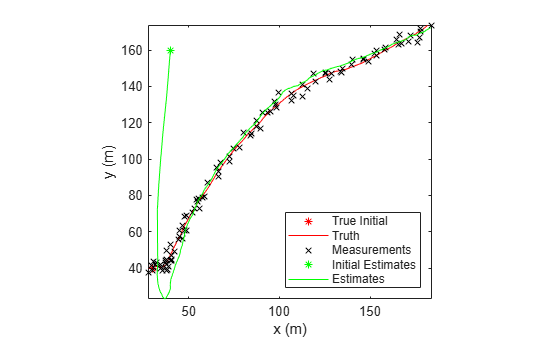## Linear Kalman Filters

Kalman filters track an object using a sequence of detections or measurements to estimate the state of the object based on the motion model of the object. In a motion model, state is a collection of quantities that represent the status of an object, such as its position, velocity, and acceleration. An object motion model is defined by the evolution of the object state.

The linear Kalman filter (`trackingKF`) is an optimal, recursive algorithm for estimating the state of an object if the estimation system is linear and Gaussian. An estimation system is linear if both the motion model and measurement model are linear. The filter works by recursively predicting the object state using the motion model and correcting the state using measurements.

### Motion Model

For most types of objects tracked in the toolbox, the state vector consists of one-, two-, or three-dimensional positions and velocities.

Consider an object moving in the x-direction at a constant acceleration. You can write the equation of motion, using Newtonian equations, as:

`$\begin{array}{l}m\stackrel{¨}{x}=f\\ \stackrel{¨}{x}=\frac{f}{m}=a\end{array}$`

Furthermore, if you define the state as:

`$\begin{array}{l}{x}_{1}=x\\ {x}_{2}=\stackrel{˙}{x},\end{array}$`

you can write the equation of motion in state-space form as:

`$\frac{d}{dt}\left[\begin{array}{c}{x}_{1}\\ {x}_{2}\end{array}\right]=\left[\begin{array}{cc}0& 1\\ 0& 0\end{array}\right]\left[\begin{array}{c}{x}_{1}\\ {x}_{2}\end{array}\right]+\left[\begin{array}{c}0\\ 1\end{array}\right]a$`

In most cases, a motion model does not fully model the motion of an object, and you need to include the process noise to compensate the uncertainty in the motion model. For the constant velocity model, you can add process noise as an acceleration term.

`$\frac{d}{dt}\left[\begin{array}{c}{x}_{1}\\ {x}_{2}\end{array}\right]=\left[\begin{array}{cc}0& 1\\ 0& 0\end{array}\right]\left[\begin{array}{c}{x}_{1}\\ {x}_{2}\end{array}\right]+\left[\begin{array}{c}0\\ 1\end{array}\right]a+\left[\begin{array}{c}0\\ 1\end{array}\right]{v}_{k}$`

Here, vk is the unknown noise perturbation of the acceleration. For the filter to be optimal, you must assume the process noise is zero-mean, white Gaussian noise.

You can extend this type of equation to more than one dimension. In two dimensions, the equation has the form:

`$\frac{d}{dt}\left[\begin{array}{c}{x}_{1}\\ {x}_{2}\\ {y}_{1}\\ {y}_{2}\end{array}\right]=\left[\begin{array}{cccc}0& 1& 0& 0\\ 0& 0& 0& 0\\ 0& 0& 0& 1\\ 0& 0& 0& 0\end{array}\right]\left[\begin{array}{c}{x}_{1}\\ {x}_{2}\\ {y}_{1}\\ {y}_{2}\end{array}\right]+\left[\begin{array}{c}0\\ {a}_{x}\\ 0\\ {a}_{y}\end{array}\right]+\left[\begin{array}{c}0\\ {v}_{x}\\ 0\\ {v}_{y}\end{array}\right]$`

The 4-by-4 matrix in this equation is the state transition matrix. For independent x- and y-motions, this matrix is block diagonal.

When you convert a continuous time model to a discrete time model, you integrate the equations of motion over the length of the time interval. In the discrete form, for a sample interval of T, the state representation becomes:

`$\left[\begin{array}{c}{x}_{1,k+1}\\ {x}_{2,k+1}\end{array}\right]=\left[\begin{array}{cc}1& T\\ 0& 1\end{array}\right]\left[\begin{array}{c}{x}_{1,k}\\ {x}_{2,k}\end{array}\right]+\left[\begin{array}{c}\frac{1}{2}{T}^{2}\\ T\end{array}\right]a+\left[\begin{array}{c}\frac{1}{2}{T}^{2}\\ T\end{array}\right]\stackrel{˜}{v}$`

where xk+1 is the state at discrete time k+1, and xk is the state at the earlier discrete time k. If you include noise, the equation becomes more complicated, because the integration of noise is not straightforward. For details on how to obtain the discretized process noise from a continuous system, See  .

You can generalize the state equation to:

`${x}_{k+1}={A}_{k}{x}_{k}+{B}_{k}{u}_{k}+{G}_{k}{v}_{k}$`

where Ak is the state transition matrix and Bk is the control matrix. The control matrix accounts for any known forces acting on the object. vk represents discretized process noise, following a Gaussian distribution of mean 0 and covariance Qk. Gk is the process noise gain matrix.

### Measurement Models

Measurements are what you observe or measure in a system. Measurements depend on the state vector, but are usually not the same as the state vector. For instance, in a radar system, the measurements can be spherical coordinates such as range, azimuth, and elevation, while the state vector is the Cartesian position and velocity. A linear Kalman filter assumes the measurements are a linear function of the state vector. To apply nonlinear measurement models, you can choose to use an extended Kalman filter (`trackingEKF`) or an unscented Kalman filter (`trackingUKF`).

You can represent a linear measurement as:

`${z}_{k}={H}_{k}{x}_{k}+{w}_{k}$`

Here, Hk is the measurement matrix and wk represents measurement noise at the current time step. For an optimal filter, the measurement noise must be zero-mean, Gaussian white noise. Assume the covariance matrix of the measurement noise is Rk .

### Filter Loop

The filter starts with best estimates of the state x0|0 and the state covariance P0|0. The filter performs these steps in a recursive loop.

1. Propagate the state to the next step using the motion equation:

`${x}_{k+1|k}={F}_{k}{x}_{k|k}+{B}_{k}{u}_{k}.$`

Propagate the covariance matrix as well:

`${P}_{k+1|k}={F}_{k}{P}_{k|k}{F}_{k}^{T}+{G}_{k}{Q}_{k}{G}_{k}^{T}.$`

The subscript notation k+1|k indicates that the corresponding quantity is the estimate at the k+1 step propagated from step k. This estimate is often called the a priori estimate. The predicted measurement at the k+1 step is

`${z}_{k+1|k}={H}_{k+1}{x}_{k+1|k}$`

2. Use the difference between the actual measurement and the predicted measurement to correct the state at the k+1 step. To correct the state, the filter must compute the Kalman gain. First, the filter computes the measurement prediction covariance (innovation) as:

`${S}_{k+1}={H}_{k+1}{P}_{k+1|k}{H}_{k+1}^{T}+{R}_{k+1}$`

Then, the filter computes the Kalman gain as:

`${K}_{k+1}={P}_{k+1|k}{H}_{k+1}^{T}{S}_{k+1}^{-1}$`

3. The filter corrects the predicted estimate by using the measurement. The estimate, after correction using the measurement zk+1, is

`${x}_{k+1|k+1}={x}_{k+1|k}+{K}_{k+1}\left({z}_{k+1}-{z}_{k+1|k}\right)$`

where Kk+1 is the Kalman gain. The corrected state is often called the a posteriori estimate of the state, because it is derived after including the measurement.

The filter corrects the state covariance matrix as:

`${P}_{k+1|k+1}={P}_{k+1|k}-{K}_{k+1}{S}_{k+1}{K}_{k+1}^{T}$`

This figure summarizes the Kalman loop operations. Once initialized, a Kalman filter loops between prediction and correction until reaching the end of the simulation.### Built-In Motion Models in `trackingKF`

When you only need to use the standard 1-D, 2-D, or 3-D constant velocity or constant acceleration motion models, you can specify the `MotionModel` property of `trackingKF` as one of these:

• `"1D Constant Velocity"`

• `"1D Constant Acceleration"`

• `"2D Constant Velocity"`

• `"2D Constant Acceleration"`

• `"3D Constant Velocity"`

• `"3D Constant Acceleration"`

To customize your own motion model, specify the `MotionModel` property as `"Custom"`, and then specify the state transition matrix in the `StateTransitionModel` property of the filter.

For the 3-D constant velocity model, the state equation is:

`$\left[\begin{array}{c}{x}_{k+1}\\ {v}_{x,k+1}\\ {y}_{k+1}\\ {v}_{y,k+1}\\ {z}_{k+1}\\ {v}_{z,k+1}\end{array}\right]=\left[\begin{array}{cccccc}1& T& 0& 0& 0& 0\\ 0& 1& 0& 0& 0& 0\\ 0& 0& 1& T& 0& 0\\ 0& 0& 0& 1& 0& 0\\ 0& 0& 0& 0& 1& T\\ 0& 0& 0& 0& 0& 1\end{array}\right]\left[\begin{array}{c}{x}_{k}\\ {v}_{x,k}\\ {y}_{k}\\ {v}_{y,k}\\ {z}_{k}\\ {v}_{z,k}\end{array}\right]$`

For the 3-D constant acceleration model, the state equation is:

`$\left[\begin{array}{c}{x}_{k+1}\\ {v}_{x,k+1}\\ {a}_{x,k+1}\\ {y}_{k+1}\\ {v}_{y,k+1}\\ {a}_{y,k+1}\\ {z}_{k+1}\\ {v}_{z,k+1}\\ {a}_{z,k+1}\end{array}\right]=\left[\begin{array}{ccccccccc}1& T& \frac{1}{2}{T}^{2}& 0& 0& 0& 0& 0& 0\\ 0& 1& T& 0& 0& 0& 0& 0& 0\\ 0& 0& 1& 0& 0& 0& 0& 0& 0\\ 0& 0& 0& 1& T& \frac{1}{2}{T}^{2}& 0& 0& 0\\ 0& 0& 0& 0& 1& T& 0& 0& 0\\ 0& 0& 0& 0& 0& 1& 0& 0& 0\\ 0& 0& 0& 0& 0& 0& 1& T& \frac{1}{2}{T}^{2}\\ 0& 0& 0& 0& 0& 0& 0& 1& T\\ 0& 0& 0& 0& 0& 0& 0& 0& 1\end{array}\right]\left[\begin{array}{c}{x}_{k}\\ {v}_{x,k}\\ {a}_{x,k}\\ {y}_{k}\\ {v}_{y,k}\\ {a}_{y,k}\\ {z}_{k}\\ {v}_{z,k}\\ {a}_{z,k}\end{array}\right]$`

### Example: Estimate 2-D Target States Using `trackingKF`

Initialize Estimation Model` `

Specify an initial position and velocity for a target that you assume moving in 2-D. The simulation lasts 20 seconds with a sample time of 0.2 seconds.

```rng(2021); % For repeatable results dt = 0.2; % seconds simTime = 20; % seconds tspan = 0:dt:simTime; trueInitialState = [30; 2; 40; 2]; % [x;vx;y;vy] processNoise = diag([0; 1; 0; 1]); % Process noise matrix```

Create a measurement noise covariance matrix, assuming that the target measurements consist of its position states.

`measureNoise = diag([4 4]); % Measurement noise matrix`

The matrix specifies a standard deviation of 2 meters in both the x- and y-directions.

Preallocate variables in which to save estimation results.

```numSteps = length(tspan); trueStates = NaN(4,numSteps); trueStates(:,1) = trueInitialState; estimateStates = NaN(size(trueStates));```

Obtain True States and Measurements

Propagate the constant velocity model, and generate the measurements with noise.

```F = [1 dt 0 0; 0 1 0 0; 0 0 1 dt; 0 0 0 1]; H = [1 0 0 0; 0 0 1 0]; for i = 2:length(tspan) trueStates(:,i) = F*trueStates(:,i-1) + sqrt(processNoise)*randn(4,1); end measurements = H*trueStates + sqrt(measureNoise)*randn(2,numSteps);```

Plot the true trajectory and the measurements.

```figure plot(trueStates(1,1),trueStates(3,1),"r*",DisplayName="True Initial") hold on plot(trueStates(1,:),trueStates(3,:),"r",DisplayName="Truth") plot(measurements(1,:),measurements(2,:),"kx",DisplayName="Measurements") xlabel("x (m)") ylabel("y (m)") axis image```Initialize Linear Kalman Filter

Initialize the filter with a state of `[40; 0; 160; 0]`, which is far from the true initial state. Normally, you can use the initial measurement to construct an initial state as `[measurements(1,1);0 ; measurements(2,1); 0]`. Here, you use an erroneous initial state, which enables you to test if the filter can quickly converge on the truth.

```filter = trackingKF(MotionModel="2D Constant Velocity",State=[40; 0; 160;0], ... MeasurementModel=H,MeasurementNoise=measureNoise)```
```filter = trackingKF with properties: State: [4x1 double] StateCovariance: [4x4 double] MotionModel: '2D Constant Velocity' ProcessNoise: [2x2 double] MeasurementModel: [2x4 double] MeasurementNoise: [2x2 double] MaxNumOOSMSteps: 0 EnableSmoothing: 0 ```
` estimateStates(:,1) = filter.State;`

Run Linear Kalman Filter and Show Results

Run the filter by recursively calling the `predict` and `correct` object functions. From the results, the estimates converge on the truth quickly. In fact, the linear Kalman filter has an exponential convergence speed.

```for i=2:length(tspan) predict(filter,dt) estimateStates(:,i) = correct(filter,measurements(:,i)); end plot(estimateStates(1,1),estimateStates(3,1),"g*",DisplayName="Initial Estimates") plot(estimateStates(1,:),estimateStates(3,:),"g",DisplayName="Estimates") legend(Location="southeast")```